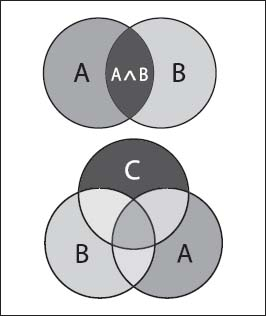NextPrevious

# What are some examples of Venn diagrams?

Venn diagrams are schematic illustrations used in logic theory to show collections of sets and the relationship between them. Overlapping circles represent the sets (or the subjects and predicates in syllogistic logic); the standard way of presenting such diagrams include the intersection of two (order-two diagram) to three (order-three diagram) circles. Based on what circles intersect and the areas shaded, a conclusion about the sets may then be read directly from the diagram. Such illustrations can include the union of two sets, the intersection of two sets, the complement of a set, and the complement of the union of two sets. (For more information about sets, see “Set Theory,” below.)In these examples of Venn diagrams, the top illustration represents an order-two diagram, and the bottom is an order-three diagram.

Close

This is a web preview of the "The Handy Math Answer Book" app. Many features only work on your mobile device. If you like what you see, we hope you will consider buying. Get the App